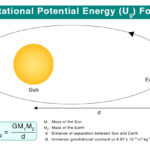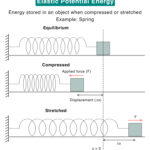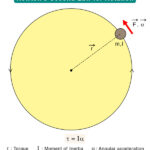Home / Physics / Planck’s Constant

# Planck’s Constant

The Planck constant is a physical constant that characterizes the mathematical equations of quantum mechanics. It describes the behavior of waves and particles, including that of light.

## History

Max Planck coined the term h in 1900 in his calculations for the distribution of energy radiated by a black body, that is, a perfect absorber of radiant energy. According to the said German physicist, light is transmitted, emitted, or absorbed in the form of discrete packets of energy called quanta. In some respects, the quantum of light behaves as an electromagnetic wave whereas, in others, it is an electrically neutral particle. This was eventually known as the photon, a term applied to the quanta of other members of the electromagnetic spectrum such as X-rays, and gamma rays as well.

On the basis of his work on this, Planck formulated a law, known as Planck’s radiation law that describes the spectral energy distribution of the electromagnetic radiation emitted by a black body at a definite temperature. Planck also determined the value of the Boltzmann constant from this derivation.

## Mathematical Formulations

The energy of each photon is h times the frequency of light. Mathematically, if we denote its energy as E, and its frequency as ʋ, then the Planck-Einstein relation says,

E=hʋ                                                                                                                                     —(i)

This is an extremely small amount of energy. Now, the wavelength λ, frequency ʋ, and the speed of light c are connected by the following formula.

ʋ=Therefore, the Planck-Einstein relation can be rewritten as,

E=This is another equation involving Planck’s constant. Now the de Broglie wavelength of any particle is associated with its linear momentum p by Planck’s constant as given by the below equation.

λ=## Reduced Planck’s Constant

In the calculations involving rotational motion, it is natural to use the angular frequency ω instead of the linear frequency ʋ. In those cases, it is useful to reduce Planck’s constant by a factor of 2π. The resulting constant is called the Dirac constant, the rationalized Planck Constant, or the Reduced Planck constant denoted by ħ (pronounced as ‘h-bar’). Hence,

ħ=Since ω=2πʋ, therefore the energy of a photon with angular frequency ω is given by,

E=ħω

Utilizing equation (i). On the other hand, the linear momentum p can be expressed as,

p=ħk

Where k is the wavenumber or the spatial frequency of the wave. It is the number of waves that reside over a particular distance.

In 1923, the Planck-Einstein relationship was generalized by French physicist and Nobel laureate Louis de Broglie. According to him, not only light but all matter could exhibit wave-like behavior, following the concept of wave-particle duality. Hence, Planck’s constant holds good for not only the quantum wavelength of photons but also that of any particle. This postulate, verified by experiments soon after, is valid throughout the quantum theory.

## Planck’s Constant Value

Its value depends on the system of units chosen. In the SI system, the value of h is 6.62607004 ×10-34 Joule second. In the electron-volts unit, it has a value of 4.135667662 x 10-15 eV seconds. In the CGS system, its value is 6.6261 × 10-27 erg second. It has the dimension of physical action, similar to that of angular momentum. In the SI system, the product of h and the speed of light in vacuum (hc) comes to be 1240 eV nm.

## Applications

Other than its significance in the quantum theory, it serves as a constant on the sub-atomic scale. Atomic unit systems are partly based on Planck’s constant. Since it is connected with only a small amount of energy, in terms of our daily experience a unit such as a mole of energy could be far more relatable. That can be calculated by multiplying the energy of a photon with the Avogadro number (approx.  6.022×1023 mol−1).

It implies that the dynamics of any physical system cannot take any arbitrary value.  It should be a multiple of a very small value, better known as the “quantum of action” or Planck’s constant. This, in turn, goes on to explain why in many cases, such as for atoms or monochromatic light, only certain energy levels are allowed, and the intermediary levels are forbidden.

Heisenberg’s Uncertainty Principle states that the momentum and the position of a particle cannot be simultaneously measured with high accuracy. It relies on Planck’s constant for its probabilistic calculations. The said constant determines the size of the confined area that can be produced by the fundamental forces to contain any particle within it. Time and energy also follow this rule.

The reduced Planck’s constant is one of the four fundamental constants in the Hartree system of atomic units. It represents the dimension of action with a numerical value equal to unity by definition. The International Committee for Weights and Measures has proposed to redefine the standard kilogram in terms of Planck’s constant, owing to its invariant nature. This attempt to derive the definition of kilogram from entirely natural sources takes the help of Einstein’s mass-energy equivalence relation (E=mc2) along with the equation (i) cited above.

The watt balance has been employed for giving such an alternative definition. The instrument can accurately measure the mass of an object by the voltage and strength of an electric current. The expression for the Rydberg constant associated with atomic spectra contains h.

## Planck Constant and the Photoelectric Effect

The photoelectric effect refers to the emission of electrons from a surface when light falls on it. These electrons are, therefore, known as photoelectrons. The renowned physicist Albert Einstein tried to explain the effect in terms of the quantization of light.

According to him, light energy is not transferred as a continuous wave, but as packets of energy or quanta which are the same as the energy elements mentioned by Max Planck in his theory. The kinetic energy of the photoelectrons (E) is directly proportional to the frequency of the incident light (f), the constant of proportionality being nothing else but Planck’s constant. This postulate that was later verified experimentally gave way to the modern version of the Planck-Einstein relation,

E=hf

He received the Nobel Prize in Physics in 1921 for his work. The work function for a particular metal, when substituted in the photoelectric equation, also allows the determination of Planck’s constant. The photoelectric equation is,

hf=ɸ + Ek

where f is the frequency of the incident light, ɸ is the work function and Eis the maximum kinetic energy of the photoelectrons. The work function of a metal is the minimum energy required to emit an electron from a surface.

Other than the multitude of significances cited above, it has provided scientists with an interesting and expanding research area. It has made theoretical physicists wonder that if the constant became zero at some point in time and space, what would be the consequences? Would it imply obvious destruction of quantization giving rise to a continuous distribution of energy? Then, at that point, quantum Physics would give way to classical Physics defining the classical limit. With Planck’s constant, the possibilities are endless. There could be more to come in the near future.

Article was last reviewed on Sunday, September 4, 2022

### Related articlesConservation of EnergyGravitational Potential EnergyElastic Potential EnergyNewton’s Second Law for Rotation